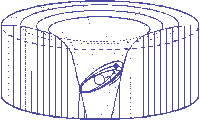Einstein's Theory of Relativity

## Classical Mechanics

by   Paul Marmet
Chapter Nine
Simultaneity and Absolute Velocity of Light.
9.1 - Simultaneity versus Identical Clock Displays.
The problem of simultaneity has been much studied in relativity. According to Einstein, simultaneous events in one frame cannot be simultaneous in another. This is known as Einstein's principle of relativity of simultaneity.
When two events take place at the same time, we say that they are simultaneous. We know that Einstein always considered that time is what clocks show. Therefore when he writes that two events are simultaneous in two different frames, he means that they occur at the moment when the clocks of observers in both frames show the same display. Since we understand that time does not flow more slowly because clocks run more slowly, Einstein's statement brings much confusion. Instead of saying that two events simultaneous in one frame are not simultaneous in another, he should have said that there is no identity of clock displays between clocks in different frames. Two clocks moving independently at different velocities do not maintain identical clock displays after a time interval. This means that even if both observers see the events at the same absolute time they will record different clock displays. Einstein's relativity of simultaneity becomes understandable only if he means that the clocks can show different displays at one given time.

9.2 - Thought Experiment on Clocks Synchronization.
In order to study this problem in more detail, let us consider figure 9.1 illustrating Einstein's thought experiment.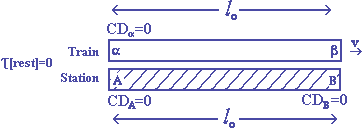Figure 9.1

Identical clocks labeled A and B are located at rest at each end of a station A-B having a length lo[rest]. There is no gradient of gravitational potential in this experiment. In front of the station A-B, a moving train a-b has a length such that when in motion, the clock labeled a located at one end of the train passes in front of clock A at the same time as clock b, located at the other end of the train, passes in front of clock B. Clocks a, b, A and B were built identically on the station. Clocks a and b were later put in motion. The synchronization of the clocks is described below.

9.3 - Synchronization of Clocks A and B.

9.3.1 - Method #1.
Clocks A and B on the station are synchronized in the following way. A pulse of light is emitted from A and reflected on a mirror at B toward A. The observer in A records on his clock a difference of clock displays DCDA for the return trip of the light.
When the traveling clock a passes near A, we arbitrarily synchronize clocks a and A together at zero. At that moment, the absolute time t[rest] on the frames is defined as zero:

 t[rest] = 0 and CDA = CDa = 0 9.1
In the second part of the experiment, a pulse of light emitted by A is received at B. At that moment, the observer at B synchronizes his clock at: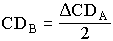9.2
Of course the absolute time is the same everywhere. This synchronization method gives a clock display on clock B equal to zero when time t[rest] equals zero:
 t[rest] = 0 when CDB = 0 9.3
The synchronization of clock b at time t[rest] = 0 will be determined in section 9.5.

9.3.2 - Method #2.
Nobody ever proved experimentally that the velocity of light is the same when moving from A to B than when moving from B to A. Michelson's experiment has shown that the time taken for light to make a return trip between two points oriented in a different direction in space is the same. However, there is an error in the Michelson-Morley demonstration.  His experiment has nothing to do with the measurement of any difference of transit time during each half of the trip. Some researchers wishing to investigate more deeply this problem have realized that the method of synchronization described in section 9.3.1 is not appropriate if the velocity of light is not identical in both directions. Consequently, other methods of synchronization have been suggested in hopes of taking into account the possibility of a non constant velocity of light in different directions. A very original method  consists in using a new reference clock labeled m, which carries the display shown by A at a very small velocity e (of the order of 10-9 of the velocity of light) on the station from A to B and later from B to A. In this way, the stationary clocks A and B can be synchronized independently in each direction with the traveling clock m. This method of synchronization is quite interesting since, as we will now show, any shift of display on clock m due to its passage from A to B (or B to A) is negligible at very low velocity.
The time taken by clock m to move from A to B is:9.4
Let us compare the difference of clock displays DCDm recorded on clock m during its travel time from A to B with the difference of clock displays DCDA recorded on the stationary clock A during the same time interval. Using equation 3.10, we have: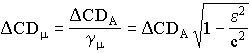9.5
The first two terms of a series expansion give:9.6
From equations 9.4 and 9.6, we have: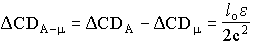9.7
Since e is very small compared with c2 (» 10-18), we can approximate e/c2 to zero. This gives:
 DCDA-m = DCDA-DCDm = 0 9.8
Consequently, clocks A and B can effectively be synchronized using a third clock m carrying the display of clock A at very low velocity from A to B. Similarly, we find that the difference of displays between clocks m and B is not significant when clock m moves from B to A. This is the result obtained when clock m moves with respect to a rest frame. In the case of clock m moving on a moving frame, the calculations will be done in section 9.7.

9.4 - Loss of Synchronization of Clock a on the Moving Frame.
Let us calculate the difference of clock displays on clock a moving across distance lo[rest] from A to B as shown on figure 9.2.Figure 9.2

Since the train moves at velocity v[rest] and the distance traveled by a is lo[rest], the time interval Dt1[rest] for clock a to reach B will be:9.9
Therefore clock a will be in front of B when:9.1
where t1 is the absolute time (after the initial synchronization) when a arrives at B.
However, the moving clock a runs at a slower rate than clock A. From equation 3.10 we find that after the time interval Dt1[rest] taken by clock a to reach point B, the display on clock a is:9.11
where gv is the value of g corresponding to velocity v. From equation 9.11, we see that even if clock a is initially synchronized with clock A (and with clock B), the synchronization is lost when a travels the distance lo[rest] (or any distance). The display of clock a becomes late with respect to clocks A and B at rest, as shown by equations 9.10 and 9.11. Let us calculate the difference of clock displays between clocks a and B when a is at B (see figure 9.2).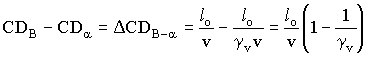9.12
The first two terms of a series expansion give:9.13
Equation 9.13 shows that in order to be compatible with the different clock rates of a and A and with the synchronization of a and A, the moving clock a must show a clock display which is different from CDB when clock a is just besides B.

9.5 - Synchronization between Moving Clocks a and b (Method #1).
In section 9.3.1, we described the synchronization of clock B with clock A. It consists in setting clock B when light is received at B, to one half of the interval DCDA taken by light to go from A to B then back to A. We now calculate the consequences of applying the same synchronization method inside a moving frame. Let us consider a pulse of light emitted from x on figure 9.3 at time t[rest] = 0. At that moment, we have:

 t[rest] = 0, CDa = CDA = CDB = 0 9.14
Let us calculate at what absolute time t2[rest] light emitted from a reaches clock b as illustrated on figure 9.3.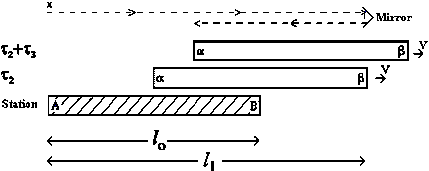Figure 9.3

We see that light approaches clock b at a relative velocity of c-v. For the observer in the moving frame, the distance to be traveled is lo[rest]. The absolute time interval Dt2[rest] to reach clock b is:9.15
When light arrives at clock b, the display on clock a is: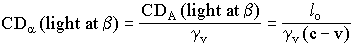9.16
After being reflected on clock b at time t2[rest], the light goes back to clock a. Since clock a and light now travel in opposite directions, light approaches clock a at a relative velocity of c+v. The absolute time interval Dt3[rest](b to a) for light to pass from b to a is: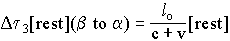9.17
Therefore the total time interval for light to travel from A to b and back to a is:
 Dt[rest](A ®b®a) = Dt2[rest](A to b)+                                                    Dt3[rest](b to a) 9.18
Using equations 9.15 and 9.17, we find:9.19
Neglecting v2 compared to c2 gives: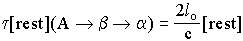9.2
Since clocks a and b are moving, their clock rate is gv times slower than the clock rate of clocks A and B. Consequently, from equation 9.20, after the return trip of light (A ®b®a) the display on clock a is: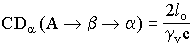9.21
Let us now synchronize clock b with clock a using method #1. Since light is emitted from a at CDa = 0, using equation 9.21, at the moment light arrives at b, clock b must be synchronized to: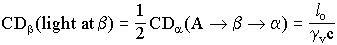9.22
However, we have seen in equation 9.16 that at the same moment, clock a shows a different display. Therefore this method of synchronization gives different clock displays at the same instant on clocks a and b. This difference is given by equations 9.16 and 9.22:9.23
Therefore at t[rest] = 0 (when CDa = 0) clock b must not be synchronized to the same display as clock a. Using equation 9.23, synchronization method #1 shows that at t[rest] = 0 we must have:9.24
The phenomenon calculated in equation 9.24 is required for a complete explanation of the mechanism of the advance of the perihelion of Mercury as mentioned in section 5.6.

9.6 - Asymmetric Relative Velocity of Light.
We have seen that the time interval Dt2[rest] (equation 9.15) for light to go from a to b is larger that the time interval Dt3[rest] (equation 9.17) for the return from b to a. However, the locations a and b between which light moves, are always separated by the constant distance lo[rest].
Because we used the synchronization method #1 on clocks a and b, the differences of clock displays recorded on those local clocks when light travels from a to b and from b to a are identical. Consequently, Einstein's synchronization method leads to a difference of synchronization between clocks a and b such that it prevents the moving observer from being able to detect that the absolute time for light to move from a to b is different from the time to move from b to a. It is this difference of synchronization between clocks a and b that prevents the observers in a and b to realize that the light that approaches them has a relative velocity different from c. The expression "velocity of light" is too vague. It is much more significant to describe the velocity at which light approaches an observer or recedes from him. Using that description, the velocity of light with respect to an observer can be different from c.
We see that this constant number representing the absolute velocity of light in any frame (in [frame] units) is just a mathematical illusion. We have shown that it is due to the different clock rate on the moving frame and to the clock synchronization of the moving observer. In fact, the velocity of light is an absolute constant in an absolute frame at rest but due to the different clock rate on the moving frame and to the synchronization, it appears constant in any frame.
One must conclude that inside a moving frame, a difference of clock displays always exists at one given instant between two clocks (a and b) located on that frame. Consequently, synchronization method #1 inside a moving frame satisfies the condition of an apparent constant velocity of light inside that frame but leads to a different setting of clocks a and b at one instant. In fact everything appears the same in the moving frame as everywhere else because the local parameters change in the exact same way to make it appear so. We will show that this apparent absoluteness of parameters within individual frames also appears when other synchronization methods are used. One can say that the observer is fooled whatever technique he uses to detect his motion.

9.7 - Synchronization of Clocks a and b (Method #2).
We have seen in sections 9.5 and 9.6 that inside the moving frame, synchronization method #1 does not lead (at a given time t[rest]) to the same clock display on clocks a and b, even if they are attached to the same frame. A moving observer might believe that he could detect this difference of clock displays using synchronization method #2 which consists in moving a third clock m at low velocity from a to b. We have seen in section 9.3.2 that there is no drift of clock display on clock m when it moves slowly across a frame at rest from A to B. Let us study now what happens when we move clock m within the moving frame a-b.Figure 9.4

Figure 9.4 illustrates a train moving at velocity v with respect to the station. Its length is lo[rest]. Clock m inside the train moves at a very small velocity with respect to the train (using rest units). The observer on the station measures the velocity of clock m to be e[rest] larger than the velocity v[rest] of the train. The total velocity u[rest] of clock m with respect to the station is then:

 u[rest] = v[rest]+e[rest] 9.25
Let us calculate the time interval Dt[rest] for clock m to move from a to b. Inside the train, clock m must travel the moving distance lo[rest] at a relative velocity of e[rest]. The time interval Dt4[rest] for clock m to travel across the moving distance lo[rest] is: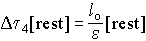9.26
The distance l2[rest] traveled by the train during that time interval Dt4[rest] is:9.27
The total distance l3[rest] traveled by clock m is then:
 l3[rest] = l2[rest] + lo[rest] 9.28
The difference of clock displays on clock a traveling distance l2[rest] is: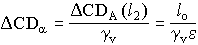9.29
where DCDA(l2) is the difference of clock displays on clock A (or B) corresponding to Dt4[rest]. The difference of clock displays on clock m travelling lo[rest] aboard the train is: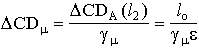9.3
where gm is the value of g corresponding to the velocity v+e of clock m. The difference of clock displays between clock a (or b) and clock m is, using equations 9.29 and 9.30: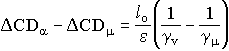9.31
Using the first two terms of series expansions we find: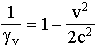9.32
and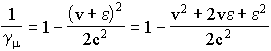9.33
Equations 9.32 and 9.33 give, to the first order: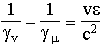9.34
Therefore, the difference between the DCDm on the moving clock inside the train and DCDa on the clock moving with the train is:9.35
We see that the difference of clock displays DDCDa-m given by equation 9.35 is directly proportional (first order) to the velocity v of the train independently of the velocity e of clock m. Consequently, a slow moving clock m inside a moving train is submitted to a slowdown of its clock rate so that when reaching clock b, its display is no longer the same as clock a as shown in equation 9.35. Let us compare this shift of display (due to velocity e) with the difference of clock displays between clocks a and b given in equation 9.23 due to the synchronization of a with b. We have seen in equation 9.23, that the difference of clock displays (to the first order) between clocks a and b at one given instant is:9.36
Equation 9.36 (or 9.23) is identical to equation 9.35. Consequently, the drift of clock display on clock m when moving from a to b is identical to the initial difference of synchronization between clocks a and b. When clock m arrives at b from a, supposedly carrying the display from a, its display will be identical to the display on clock b.
To study the case when clock m moves in the opposite direction, we just have to substitute v+e in equation 9.33 by v-e and replace DCDa in equation 9.31 by DCDb. This is correct because equation 9.29 gives not a clock display but a difference of clock displays. Equation 9.34 stays the same except for a negative sign and we get for 9.35: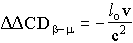9.37
We see then that when clock m moves slowly in the opposite direction from b to a, it will run at a faster rate so that when it arrives besides clock a, its display will be the same as the one already existing on clock a. We see that clock m shows the display of clock a when located near a and the display of clock b when located near b. One must conclude that synchronization method #2 is totally unable to reveal the difference of clock displays between a and b inside a moving frame generated by synchronization method #1.

9.8 - References.
 This method is often used by F. Selleri, Universita di Bari, Dipartimento di Fisica, Sezione, INFN, Via Amendola, 173, I70126 Bari, Italy.

9.9 - Symbols and Variables.

 CDA clock display on clock A CDa clock display on clock a CDB clock display on clock B CDb clock display on clock b lo[rest] length of the station and the moving train in rest units t[rest] absolute time (in rest units)
<><><><><><><><><><><><>

Chapter8 ContentsChapter 10